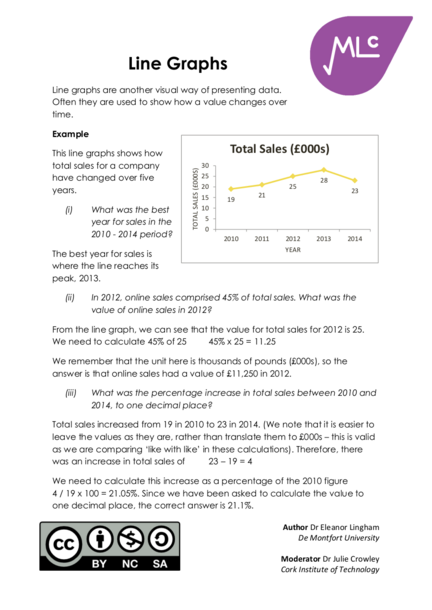# Line GraphsContributed by:This pdf shows that the Line graphs are another visual way of presenting data. Often they are used to show how a value changes over time.
1. Line Graphs
Line graphs are another visual way of presenting data.
Often they are used to show how a value changes over
This line graphs shows how Total Sales (£000s)
total sales for a company 30
TOTAL SALES (£000S)
have changed over five 25
28
20 25
years. 21
23
15 19
(i) What was the best 10
5
year for sales in the
0
2010 - 2014 period? 2010 2011 2012 2013 2014
YEAR
The best year for sales is
where the line reaches its
peak, 2013.
(ii) In 2012, online sales comprised 45% of total sales. What was the
value of online sales in 2012?
From the line graph, we can see that the value for total sales for 2012 is 25.
We need to calculate 45% of 25 45% x 25 = 11.25
We remember that the unit here is thousands of pounds (£000s), so the
answer is that online sales had a value of £11,250 in 2012.
(iii) What was the percentage increase in total sales between 2010 and
2014, to one decimal place?
Total sales increased from 19 in 2010 to 23 in 2014. (We note that it is easier to
leave the values as they are, rather than translate them to £000s – this is valid
as we are comparing ‘like with like’ in these calculations). Therefore, there
was an increase in total sales of 23 – 19 = 4
We need to calculate this increase as a percentage of the 2010 figure
4 / 19 x 100 = 21.05%. Since we have been asked to calculate the value to
one decimal place, the correct answer is 21.1%.
Author Dr Eleanor Lingham
De Montfort University
Moderator Dr Julie Crowley
Cork Institute of Technology
2. Line graphs can also be used to show how two or more
values change over time.
This line graph shows share
price changes to two Share Price
companies over a four
£9.00
year period. £8.50
£8.00
(i) What is the
SHARE PRICE
£7.50
difference £7.00
ABC Ltd
between the £6.50
DEF Plc
ABC Ltd share £6.00
£5.50
price and the
£5.00
DEF Plc share 2012 2013 2014 2015
price in 2012? YEAR
The difference between
the share prices in 2012 is 8.20 – 7.50 = 0.70
There is a difference of £0.70 between the ABC Ltd share price and the DEF
Plc share price in 2012.
(ii) In which year was the largest percentage increase in share price for
ABC Ltd?
From the line graph, we can see that the ABC Ltd share price increased in
2014 and 2015. We need the calculate the increase in price in each year
2014 6.50 – 6.00 = 0.50
2015 6.70 – 6.50 = 0.20
We then calculate each as a percentage the Speed Tip!
share price in the earlier year
From the graph, you
0.50 / 6.00 x 100 = 8.33%
could guess the
0.20 / 6.50 x 100 = 3.07%